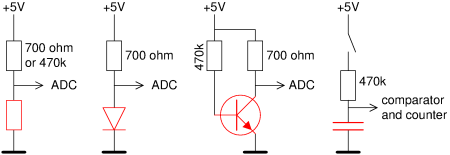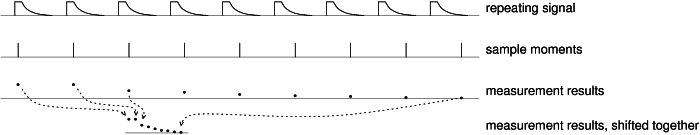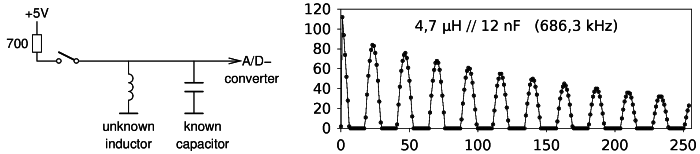# Measuring small inductances and capacitance with the "AVR transistortester"

Pieter-Tjerk de Boer, PA3FWM pa3fwm@amsat.org

(This is an adapted version of part of an article that I wrote for the Dutch amateur radio magazine Electron, December 2015.)

## The "AVR transistortester"

The so-called AVR transistortester is a handy and popular tester for many kinds of components, about which, to my surprise, little has been written in amateur radio magazines. It consists of not much more than an AVR microcontroller, an LCD display and some passive components. As the name indicates, it can test transistors, but besides that it can also deal with diodes, thyristors, triacs, resistors, capacitors, and inductors, and therefore should perhaps be called "AVR component tester". The tester figures out automatically what kind of component is connected to it and which pin is which. Furthermore, it measures some key characteristics of the component, like the current gain in case of a transistor. It was originally designed by Markus Frejek and is nowadays developed further by Karl-Heinz Kübbeler (see here). It can easily be homebuilt, but is also sold ready-built, among others on Ebay and at radio flea markets.The left part of the figure shows the essence of the tester's circuit diagram. We see that each pin of the Device Under Test (DUT) is connected to three I/O-pins of the microcontroller: directly to one I/O-pin, via a 680 ohms resistor to another I/O-pin, and finally via 470 kiloohm to a third. Now we should know that each I/O-pin of the microcontroller can either be programmed as in an input (then it goes into a high-impedance state), or as an output; in the latter case it will be connect via a low internal resistance of about 20 ohms to 0 volts (logical 0) or 5 volts (logical 1). The result is that each pin of the DUT can either float, or via 20, 700 or 470k ohms be connected to 0 or +5 volts. Furthermore, the three I/O-pins to which the DUT is connected without resistors, are also inputs of the micrcontroller's built-in A/D converter, so the voltage on each of the DUT's pins can be measured, and of a comparator. All of this leads to the equivalent schematic shown at the right, where the switches are all controlled by the microcontroller's software.How can components be tested? That is illustrated in the next figure using a couple of examples that can be realised by the software by setting the switches in the appropriate positions. From left to right we see resistance measurement (the unknown resistor (red) forms a voltage divider with the known resistor in the tester), diode test (voltage drop across the diode is measured, 0.6 V for a conducting silicon diode, 5 V in the reverse direction), current gain of a transistor (base current is supplied via 470 k, and the resulting collector current is measured), and capacitance (after closing the switch, it is measured how long it takes for the capacitor to be charged to 1.1 V). Automatically figuring out the connections of a transistor is done by simply trying all possibilities and choosing the one which gives the largest current gain.

So, it's a very handy device, which deserves more attention in the amateur radio literature than it gets so far. However, it also has some limitations which are unhandy precisely for radio amateurs: it cannot measure inductances better than in 10 µH steps, which is rather large for HF; and also the measurement of small capacitances is limited, with a resolution of 1 pF.

## Measuring small coils and capacitances

The problem with measuring small capacitances and inductances with this tester, is that everything happens far too quickly. Just do the math: 1 pF with 470 kΩ (which is the largest available test resistor) results in an RC time of about 0.5 µs, and 10 µH with 20 Ω (the smallest available test resistor) results in an L/R time of that same order of magnitude. The A/D converter in the microcontroller needs about 100 µs for a single conversion: that's way too slow for this. As an alternative, the microcontroller's comparator can be used to measure how long it takes to reach a (fixed) threshold of 1.1 volt, with an accuracy in the order of the clock frequency; but that's not really good enough either, hence the limitations on measuring small C and L.

I wanted to improve this, and got the idea of using the sample&hold circuit which is in the microcontroller in front of the A/D converter. This circuit makes sure that although the A/D conversion takes about 100 µs, the signal being measured does not need to be available during all that time: it is "sampled", i.e., stored in analog form, and that value is converted to digital.With such an S&H one can, despite the slow A/D converter, still measure fast signals in much detail, if they are repetitive. One does this by taking a sample every time the signal repeats, but every time a little bit later, as sketched in the figure. Together, the samples still represent the original signal. This trick was already used half a century ago in oscilloscopes: with an S&H at its input, the scope could be used for signals at much higher frequencies than what the rest of the electronics in the scope could handle.

Using this, the capacitance measurement can mostly be improved by measuring many points on the (dis)charge curve, instead of only one as done with the comparator. In theory, these points should lie on an exponential curve; by "fitting" such a curve through the measured points, the time constant can be found, while averaging out errors in the individual measurements.

For the inductance measurement, this is still not good enough, because at small inductances the current simply rises to quickly: already within 1 clockcycle of the processor the final value has almost been reached, leaving little to be measured. In order to still measure small inductances, I connect a capacitor of about 22 nF in parallel, forming an LC circuit. By the applying a voltage to this LC circuit during a short time (via one of the microcontroller's I/O pins), the circuit is "excited"; from that moment on, the voltage across it is a damped sine wave, which can be measured using the sampling trick.The figure shows an example of such a measurement. We see 256 consecutive voltage measurements, made by the microcontroller, using the S&H, each time 62.5 ns (that is 1 period of the microcontroller's 16 MHz clock frequency) farther away from the moment at which the impulse is applied. We see only the positive half of a damped sine wave. The negative half is invisible because the A/D converter cannot handle negative voltages. But even with just the positive halves it is easy to determine the duration of the period, and by interpolation a resolution can be reached which is much better than the period of the microcontroller's clock. From the period the frequency can be calculated, and together with the known capacitance, the inductance follows. Furthermore, the circuit's Q factor can be determined from how quickly the amplitude decreases, and this will usually be a good indication of the inductor's Q (unless a low-quality capacitor is used).

In practice, this principle turns out to be usable for inductors as low as about 100 nH, using a test capacitor of 22 nF. At even smaller inductances, the resulting frequency becomes too high to be measured, given the microcontroller's 16 MHz clock; using an even larger capacitor would solve this, but it turns out that the resonance then becomes so weak (low Q) that the measurement fails.

This new measuring method for small capacitances and inductances that I developed, are not yet integrated in the standard software for these testers. However, I'm now working with its author (Karl-Heinz Kübbeler) to integrate my extensions into it, and improve them further; they are already available in the project's SVN server (see here).